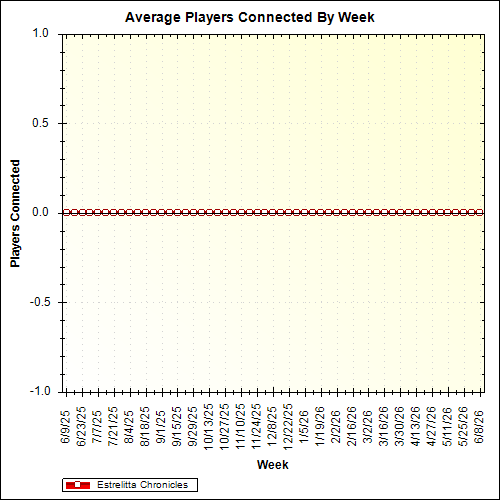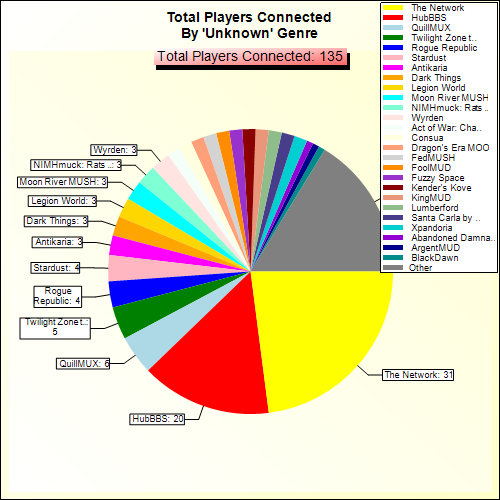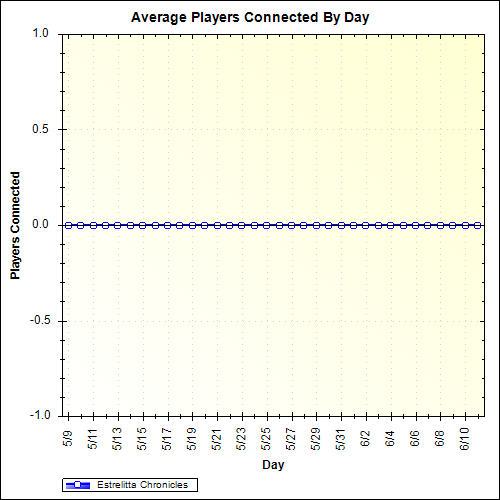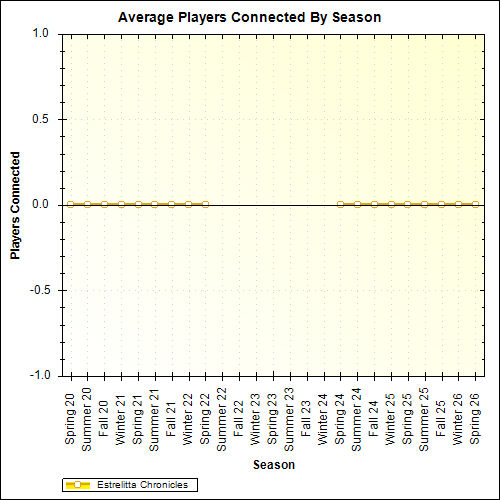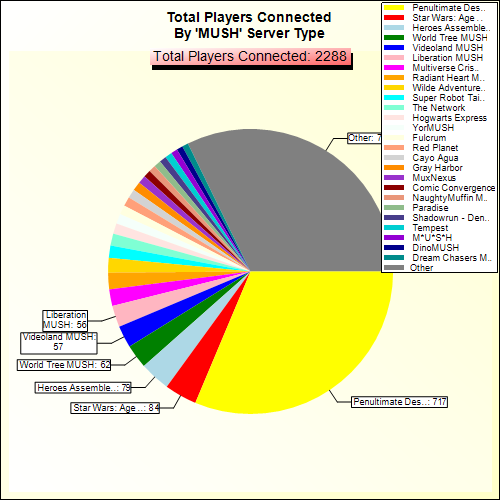Estrelitta Chronicles
Unknown-themed MUSH (Multi-User Shared Hallucination).
Ranked 682nd of 809 worlds statistically.
Ranked 40th of 45 worlds in the Unknown genre statistically.

Db Size:
175

Players Connected:
0 (46 minutes ago)

Maximum Connected:
0 (last 30 days)
Status:
UP

Version:
RhostMUSH Alpha 4.1.0RL(A)

Average Connected:
0 (last 30 days)

Minimum Connected:
0 (last 30 days)
Connection Screen
Welcome to The
Estrellita Chronicles
an RhostMUSH

And a Science Fiction Series by
written by Rick Donaldson
email Rick at: r.daledonaldson@gmail.com
Find Book One: Aftermath's Children on Amazon - Paperback and Digital!

/~\=/~\=/~\=/~\=/~\=/~\=/~\=/~\=/~\=/~\=/~\=/~\=/~\=/~\=/~\=/~\=/~\=/~\=/~\
((  To create new character     ----------=>   create <player> <password>  ))
)) To continue old character   ----------=>   connect <player> <password>((
((  To connect a guest          ----------=>   connect guest guest         ))
)) To see who is on            ----------=>   WHO                        ((
((  To quit your character      ----------=>   QUIT                        ))
)) To get help once connected  ----------=>   help (i.e. : help commands)((
\_/=\_/=\_/=\_/=\_/=\_/=\_/=\_/=\_/=\_/=\_/=\_/=\_/=\_/=\_/=\_/=\_/=\_/=\_/
~   ~   ~   ~   ~   ~   ~   ~   ~   ~   ~   ~   ~   ~   ~   ~   ~   ~   ~